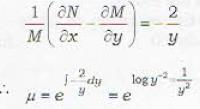Courses

# Differential Equations - 16

## 20 Questions MCQ Test Topic-wise Tests & Solved Examples for IIT JAM Mathematics | Differential Equations - 16

Description
This mock test of Differential Equations - 16 for Mathematics helps you for every Mathematics entrance exam. This contains 20 Multiple Choice Questions for Mathematics Differential Equations - 16 (mcq) to study with solutions a complete question bank. The solved questions answers in this Differential Equations - 16 quiz give you a good mix of easy questions and tough questions. Mathematics students definitely take this Differential Equations - 16 exercise for a better result in the exam. You can find other Differential Equations - 16 extra questions, long questions & short questions for Mathematics on EduRev as well by searching above.
QUESTION: 1

### The differential equation of all circles of radius a is given by

Solution:

I Proof: The family of circles of radius a is given by
(x - h)2 + (y - k)2 = a2,    .......(i)
where h and k are the parameters to be eliminated. Since there are two arbitrary constants, therefore equation (i) is to be differentiated twice and the order of the differential equation must be 2.
Now differentiating (i) twice, we get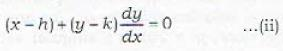and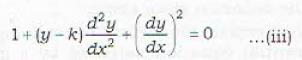Eliminating k and h from equations (i), (ii) and (iii), the required differential equation of the circles of radius a is given by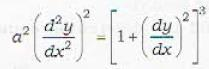QUESTION: 2

### A differential equation is said to be linear if

Solution:

Definition : A differential equation is said to be linear if.
i. the dependent variable and each of its derivatives occurs to the first degree only and
ii. no products of dependent variable and or its derivatives occur.

QUESTION: 3

### Which of the following differential equations is linear?

Solution:

Remark : Observe that the dependent variable y and its derivatives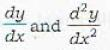occur to the first degree and there are no products of them in statement (d).
The product of y and dy/dx occurs in (a), (b) and (c)

QUESTION: 4

Which of the following differential equations is linear but not of first degree

Solution:

The differential equations in (a), (b) and (c) are of first degree but none of them is linear.

QUESTION: 5

A differential equation of first degree

Solution:

(i)(a) is not correct because a differential equation of first degree may not be linear. For example, the differential equation.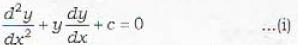is of first degree but non-linear,
(ii) (b) is not correct because a differential equation of first, degree is not necessarily of first order. The differential equation (i) is of first, degree but of second order.
(iii) (d) is not correct because a differential equation of first degree may be of first order and may not be linear.

QUESTION: 6

A linear differential equation

Solution:

Any differential equation which is not of first degree can not be linear.

QUESTION: 7

Which one of the following is a standard form of the first order differential equation of first degree?

Solution:

The differential equation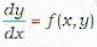is of first order and first degree. However, it may or may not be linear.
M(y, y)dx + N(x, y)dy = 0
can be rewritten as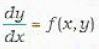and therefore is of first degree and first order. It also may or may not be linear.

QUESTION: 8

If M and N are functions of x and y, then the equation Mdx + Ndy = 0 is exact if

Solution:

The necessary and sufficient condition for the differential equation
M(x, y)dx + N(x, y)dy = 0 to be exact is that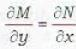Proof: Condition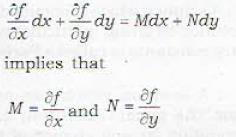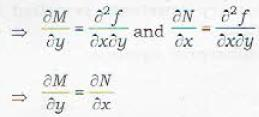Remark : An exact differential equation can be written as du = 0. where u is some function of x and y.

QUESTION: 9

The necessary and sufficient condition for the differential equation M(x, y)dx + N(x, y)dy = 0 to be exact is that

Solution:
QUESTION: 10

If P(x) and Q(y) are arbitrary functions of x and y respectively, then the differential equation P(x)dx + Q(y)dy = 0

Solution:

Proof : The given differential equation is
P(x)dx+Q(y)dy = 0 ...(i)
Comparing it with
Mdx + Ndy = 0 ...(ii)
∴ M = P(x) and N = Q(y)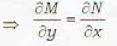is always satisfied.
⇒ D.E. (i) is always exact.

QUESTION: 11

If P(y) and Q(x) are arbitrary functions of y and a respectively, then the differential equation P(y)dx+ Q(x)dy = 0

Solution:

Proof : The given differential equation is
P(y)dx+Q(x)dy = 0 ...(i)
Comparing (i) with
Mdx + Ndy= 0 ...(ii)
M = P(y) and N = Q(x)
Differential equation (i) will be exact if and only if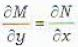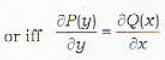...(iii)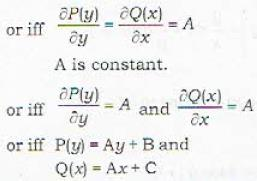QUESTION: 12

Which of the following differential equation is exact?

Solution:

Consider the differential equation in (a),
(y2 + x) dx + (y3 + x)dy = 0 ... (i)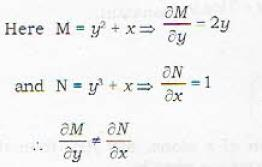so that DE (i) is not exact.
(ii) The differential equation in (b) can be rewritten as
(x + y - 1) dx - (x + y + 1 ) = 0  .....(ii)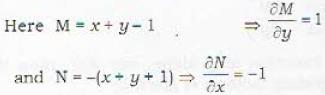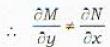so that DE (ii) is not exact
(iii) The differential equation in (c) is
2xy dx + (x + y2) dy = 0  .....(iii)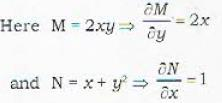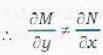so that DE (iii) is not exact.
Thus none of the differential equations in (a), (b) and (c) is exact.

QUESTION: 13

Which of the following differential equations is exact?

Solution:

The differential equation in (a) is exact.
Remark : Verify yourself that (a) is exact but (b), (c) or fd) are not exact.

QUESTION: 14

Which of the following provides a solution of the exact differential equation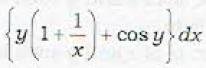+ {x + log x - x sin y}dy = 0

Solution:

The given differential equation can be written as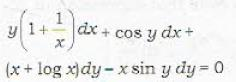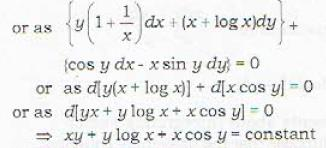QUESTION: 15

Which of the following provides a solution of the exact differential equation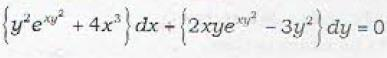Solution:

The given differential equation can be rewritten as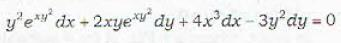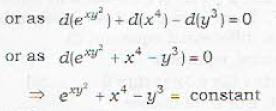QUESTION: 16

Which of the following is not an exact differential?

Solution: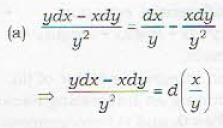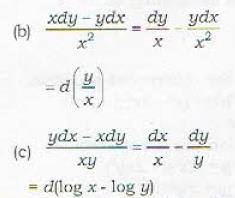∴ expressions in (a), (b) and (d; are exact.
Since the expression
xdy - ydx
in (c) can not be expressed as du for some function u of x and y, therefore the expression in (c) is not exact.

QUESTION: 17

Which of the following is an exact differential?

Solution:
QUESTION: 18

Which of the following is an exact differential?

Solution:

Since the expression in (a) can be written as an exact expression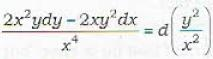QUESTION: 19

Which of the following is not an exact differential?

Solution:

The expression in (a) is not exact. Hence (a) is the correct answer.
Remark : Note that expression in (a)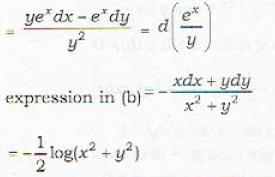Definition : Suppose that tne differential equation. M dx + N dy = 0    ...(i)
is not exact but the differential equation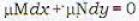is exact, where μ =  F(x, y) for a suitably chosen function F. Then μ is called an integrating factor of the differential equation (i).
Ex. : The differential equation
(3ty + 4xy2) dx + (2x + 3x2y) dy = 0    ...(ii)
is not exact. But if we choose
μ = x2 y.
then the differential equation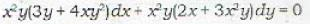becomes exact.
∴ μ = x2 y is an integrating factor of (ii).
Rules for finding an Integrating Factor :
Rule : 1: If Mx ± Ny ≠ 0. and is homogeneous in x and y then the integrating factor is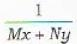Ex. : Consider the differential equation
(x2y - 2xy2)dx- (x3 - 3x2y)dy = 0    ...(iii)
verify that
(i) equation (iii) is noi exact and (ii)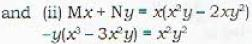Intergrating factor is given by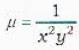Hence the equation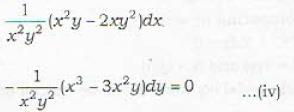should be exact. Now equation (iv) can be written as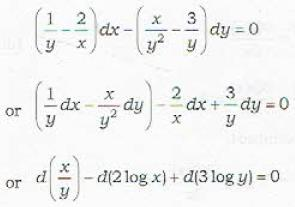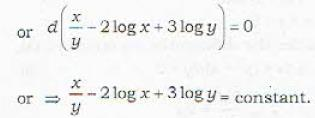Rule : 2. If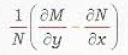is a function of x alone, say f(x), then the integrating factor μ is given by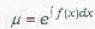Rule : 3. If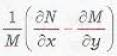is a function of y alone, say φ(y), then the integrating factor μ is given by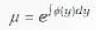QUESTION: 20

The integrating factor of the differential equation xdx - ydx = xy2dx is

Solution:

Noto that